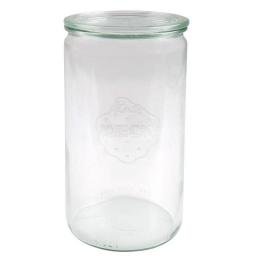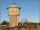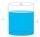# Jar

From the cylinder shaped jar after tilting spilled water so that the bottom of the jar reaches the water level accurately into half of the base. Height of jar h = 7 cm and a jar diameter D is 12 cm. How to calculate how much water remains in the jar?

Correct result:

V =  168 cm3

#### Solution:We would be pleased if you find an error in the word problem, spelling mistakes, or inaccuracies and send it to us. Thank you!Tips to related online calculators
Tip: Our volume units converter will help you with the conversion of volume units.

## Next similar math problems:

• Water reservoirThe reservoir shape of a cylinder having a diameter 8 m and a height 2.8 m. The device indicates that the reservoir contains 800 hectoliters of water. Calculate how much % of volume is used.
• Truncated coneCalculate the height of the rotating truncated cone with volume V = 1115 cm3 and a base radii r1 = 7.9 cm and r2 = 9.7 cm.
• PebbleThe aquarium with internal dimensions of the bottom 40 cm × 35 cm and a height of 30 cm is filled with two-thirds of water. Calculate how many millimeters the water level in the aquarium rises by dipping a pebble-shaped sphere with a diameter of 18 cm.
• Volume and surfaceCalculate the volume and surface area of the cylinder when the cylinder height and base diameter is in a ratio of 3:4 and the area of the cylinder jacket is 24 dm2.
• AquariumThe box-shaped aquarium is 40 cm high; the bottom has dimensions of 70 cm and 50 cm. Simon wanted to create an exciting environment for the fish, so he fixed three pillars to the bottom. They all have the shape of a cuboid with a square base. The base edg
• Cone containerRotary cone-shaped container has a volume 1000 cubic cm and a height 12 cm. Calculate how much metal we need for making this package.
• CylinderThe cylinder-shaped container has 80 liters of water. The water reaches 45 cm height. How many liters of water will be in a container if water level extend to a height 72 cm? Write the result in liters, write down only as a whole or decimal number.
• Equilateral cylinderEquilateral cylinder (height = base diameter; h = 2r) has a volume of V = 199 cm3 . Calculate the surface area of the cylinder.
• Tin with oilTin with oil has the shape of a rotating cylinder whose height is equal to the diameter of its base. Canned surface is 1884 cm2. Calculate how many liters of oil is in the tin.
• ConvaHow many liters of water fit into the shape of a cylinder with a bottom diameter 20 cm and a height 45 cm?
• Cylinder horizontallyThe cylinder with a diameter of 3 m and a height/length of 15 m is laid horizontally. Water is poured into it, reaching a height of 60 cm below the axis of the cylinder. How many hectoliters of water is in the cylinder?
• Tank and waterCylindrical tank were poured with 3.5 liters of water. If tank base diameter is 3 dm, how height is water level in?
• TrayWjat height reach water level in the tray shaped a cuboid, if it is 420 liters of water and bottom dimensions are 120 cm and 70 cm.
• Water channelThe cross section of the water channel is a trapezoid. The width of the bottom is 19.7 m, the water surface width is 28.5 m, the side walls have a slope of 67°30' and 61°15'. Calculate how much water flows through the channel in 5 minutes if the water flo
• Hemisphere cutCalculate the volume of the spherical layer that remains from the hemisphere after the 3 cm section is cut. The height of the hemisphere is 10 cm.
• Four prismsQuestion No. 1: The prism has the dimensions a = 2.5 cm, b = 100 mm, c = 12 cm. What is its volume? a) 3000 cm2 b) 300 cm2 c) 3000 cm3 d) 300 cm3 Question No.2: The base of the prism is a rhombus with a side length of 30 cm and a height of 27 cm. The heig
• ContainerThe container has a cylindrical shape the base diameter 0.8 m and the area of the base is equal to the area of the wall. How many liters of water can we pour into the container?